# Lakhmir Singh Solutions For Class 8 Science Chapter 11 Force and Pressure

Force is defined as the push or pull experienced by the object because of another object. To apply force, minimum two objects need to be involved: one which exerts force and the other that experiences the force. For example, sliding a book over the surface of the table. It is interesting to know that force can either act in the same direction or in opposite directions. When a force acts in the same direction, the net force is the sum of the magnitude of both the force. Whereas when a force acts in the opposite direction, the net force is the difference between the magnitude of both the forces. Following are the effects of force:

• Force can change the state of motion
• Force can change the shape and size of an object
• Force can change the direction of the moving object

There are two types of forces: contact force and non-contact force. Frictional force and muscular forces are the types of contact force while a magnetic force, the gravitational force, and the electrostatic force are the types of non-contact force.

The pressure is defined as the force acting per unit area of a surface. Liquids and gases are capable enough of exerting pressure on the inner walls of the container in which they are kept. The pressure exerted by the air is known as atmospheric pressure. Following is the formula of pressure:

 Pressure = (Force)/(Area)

## Download PDF Of Lakhmir Singh Science Class 8 Solutions For Chapter 11 Force and Pressure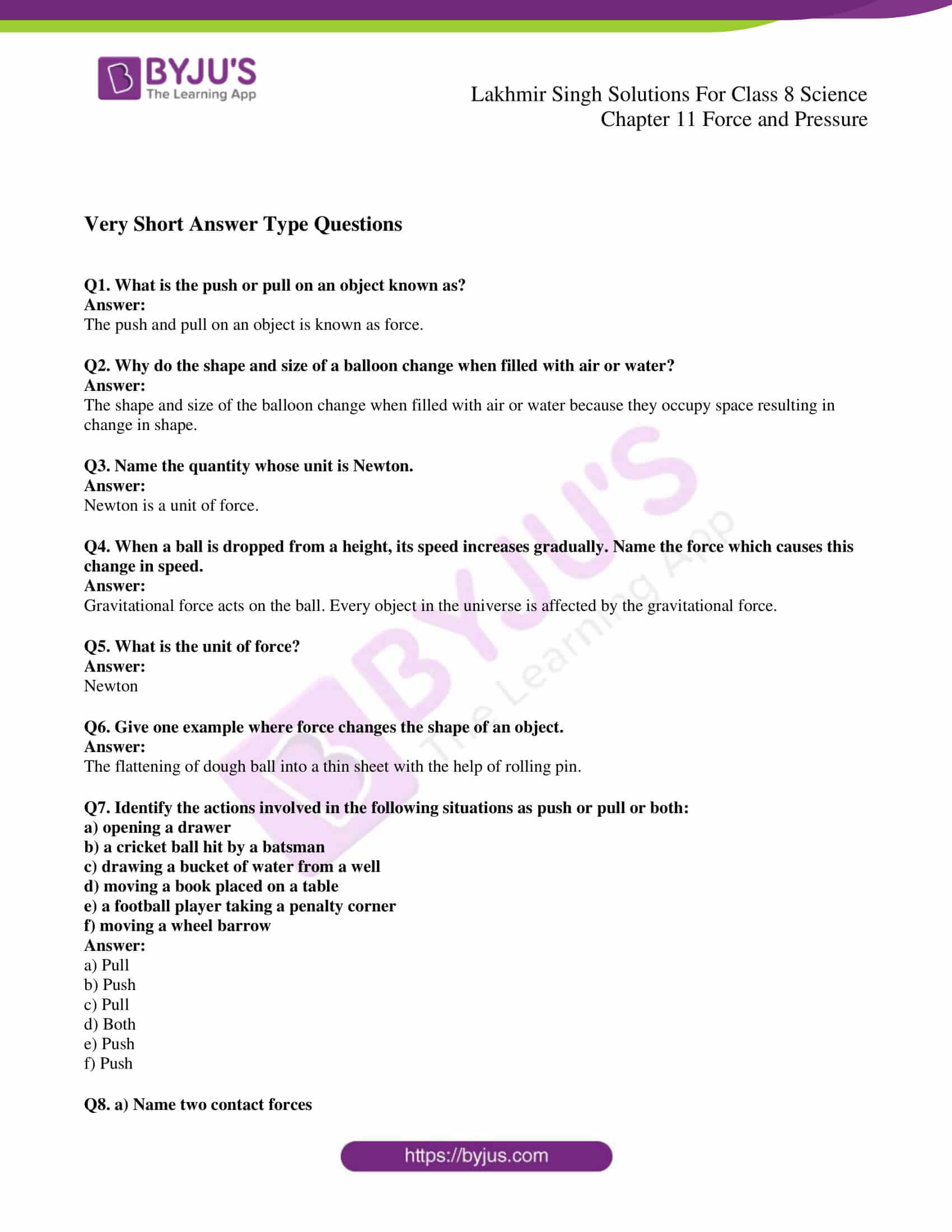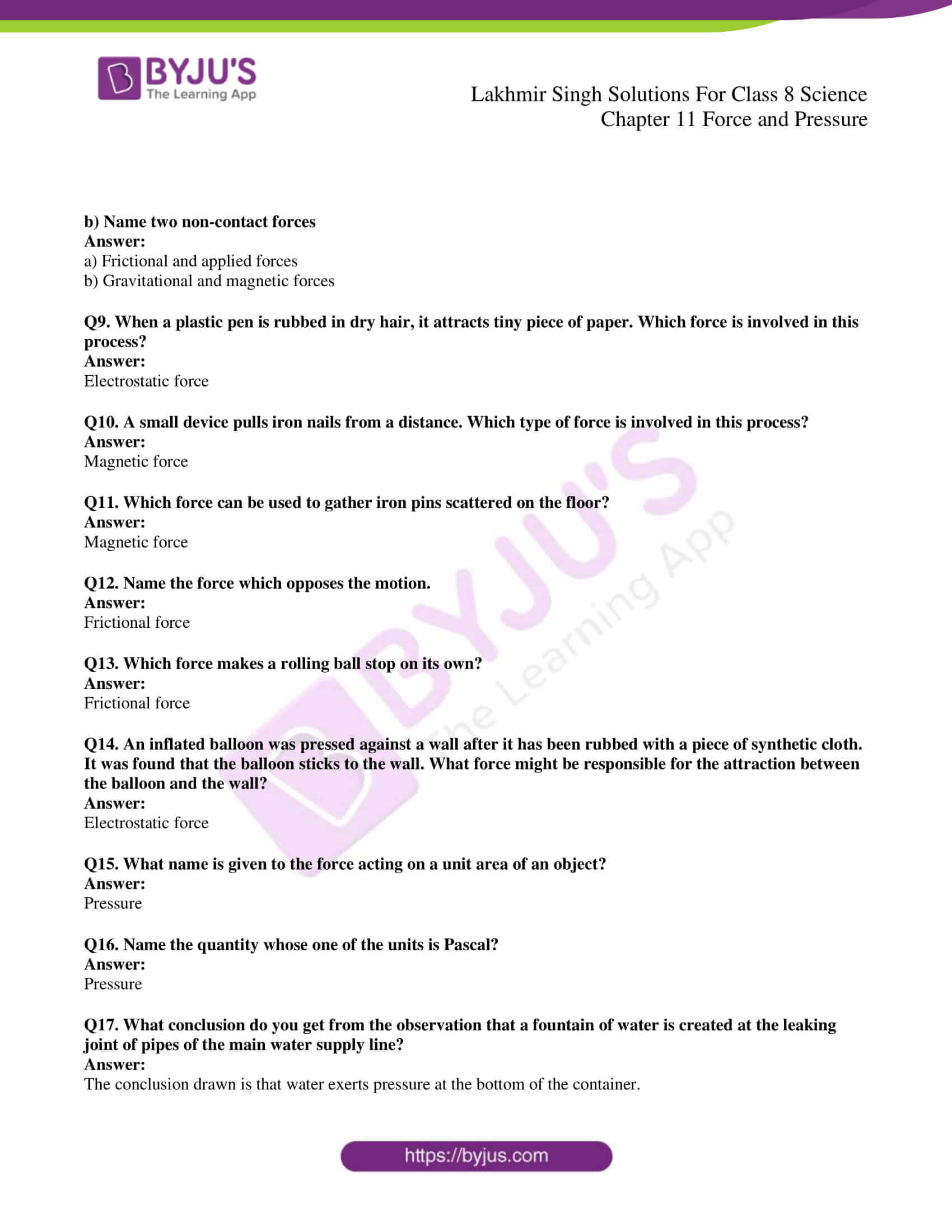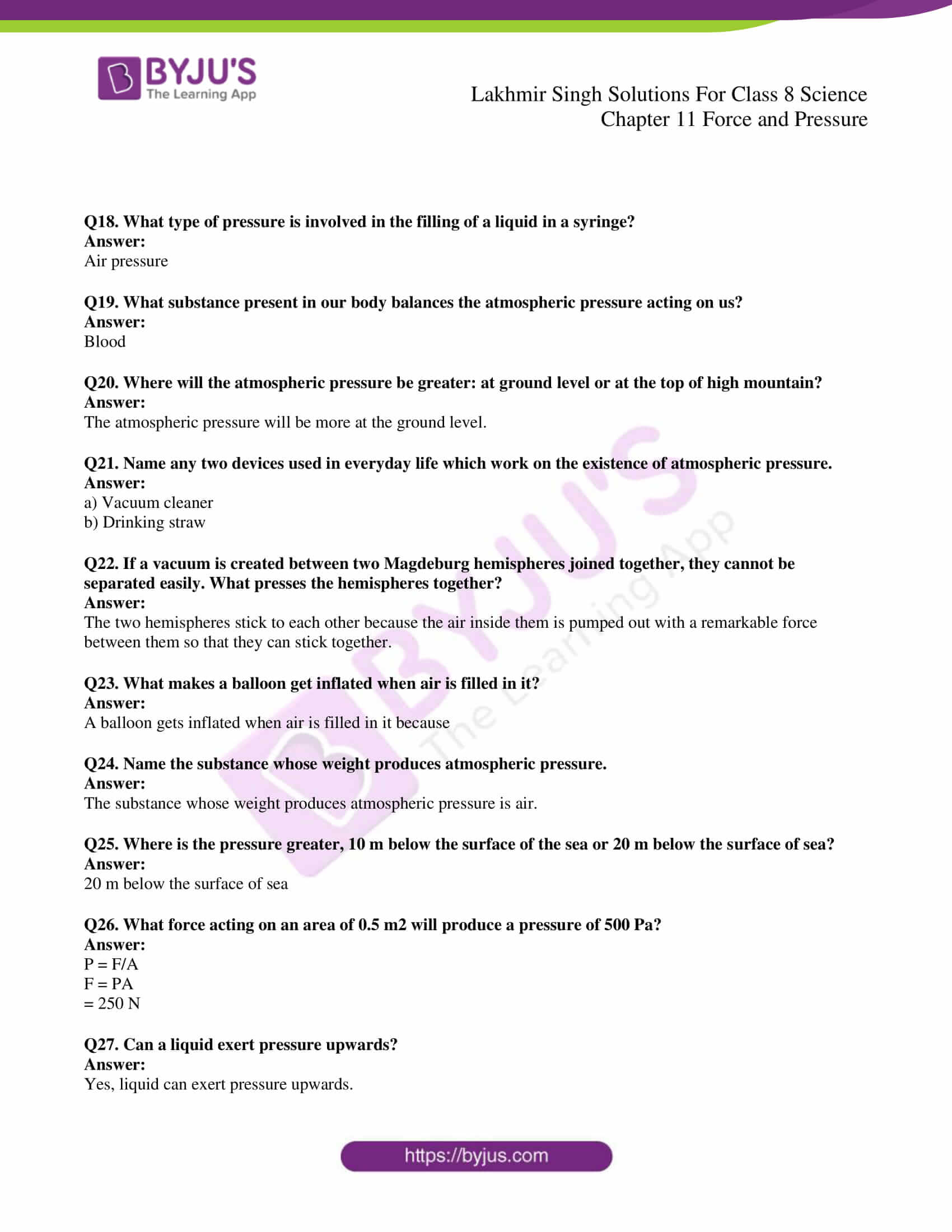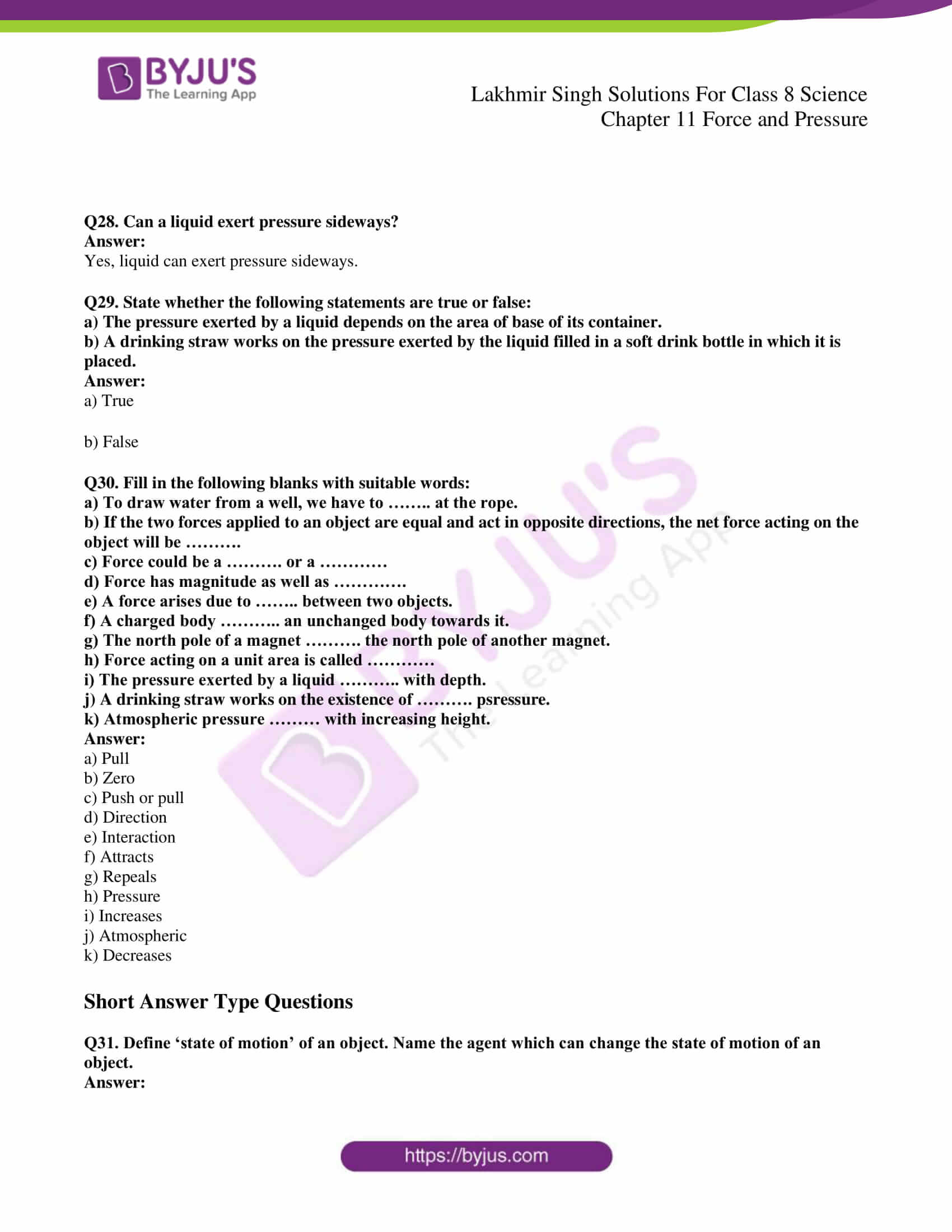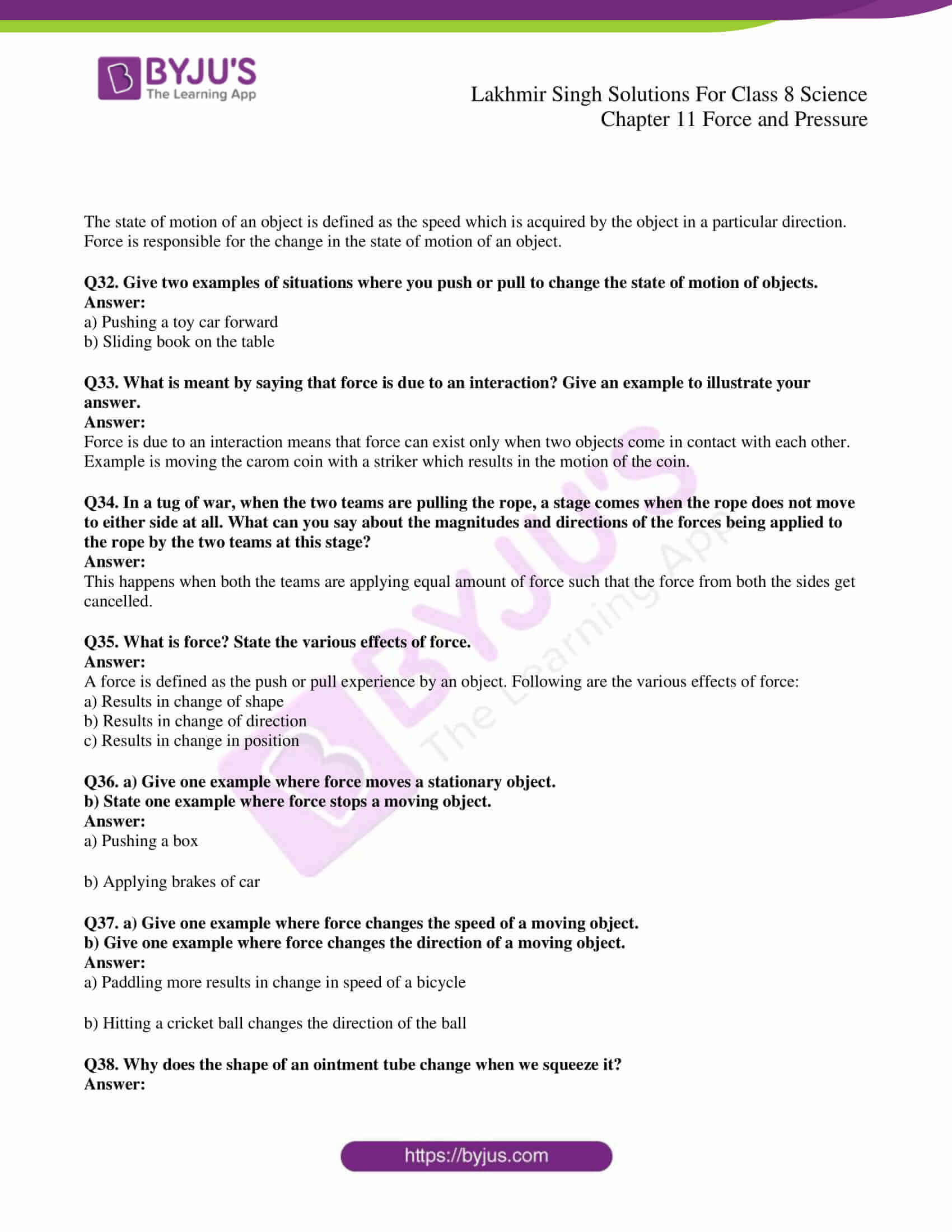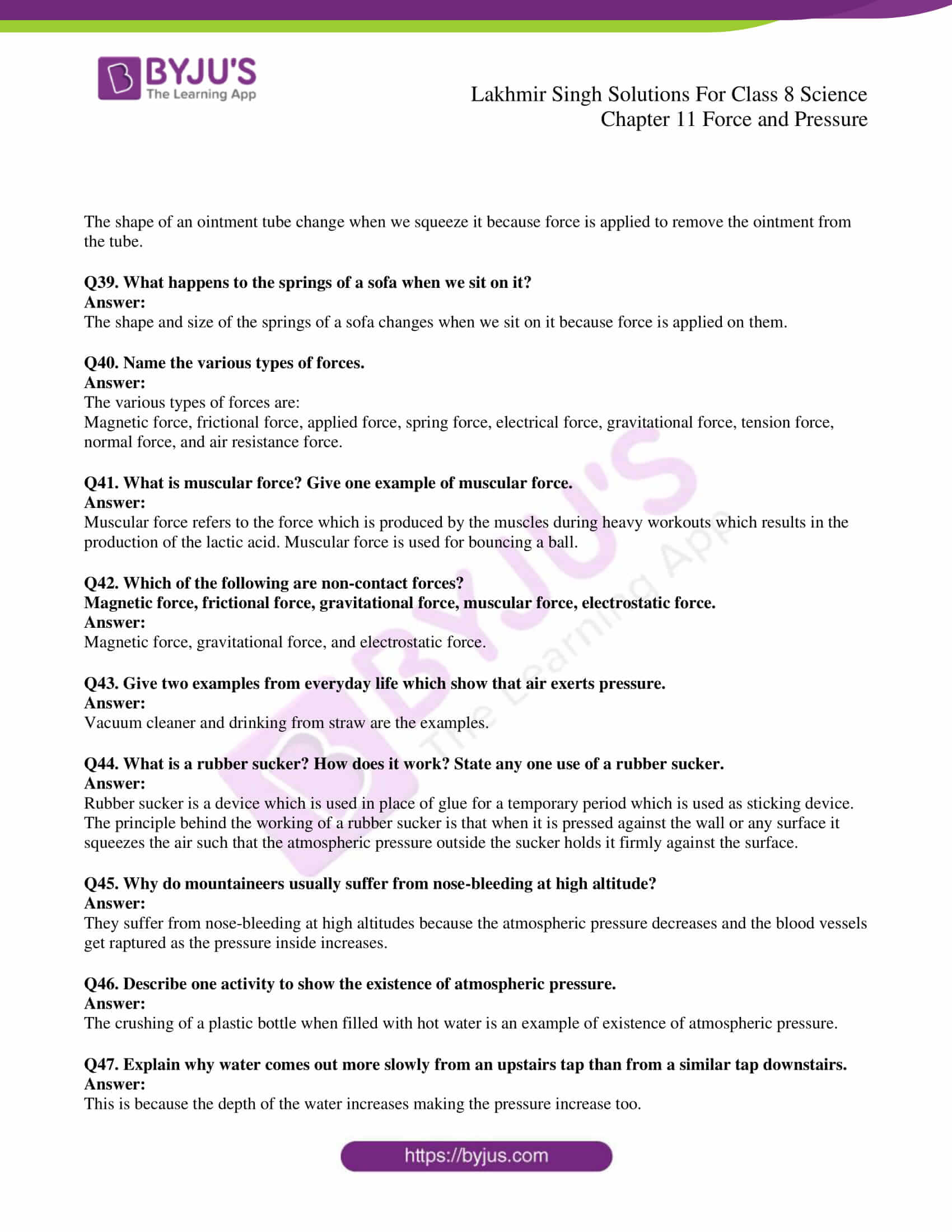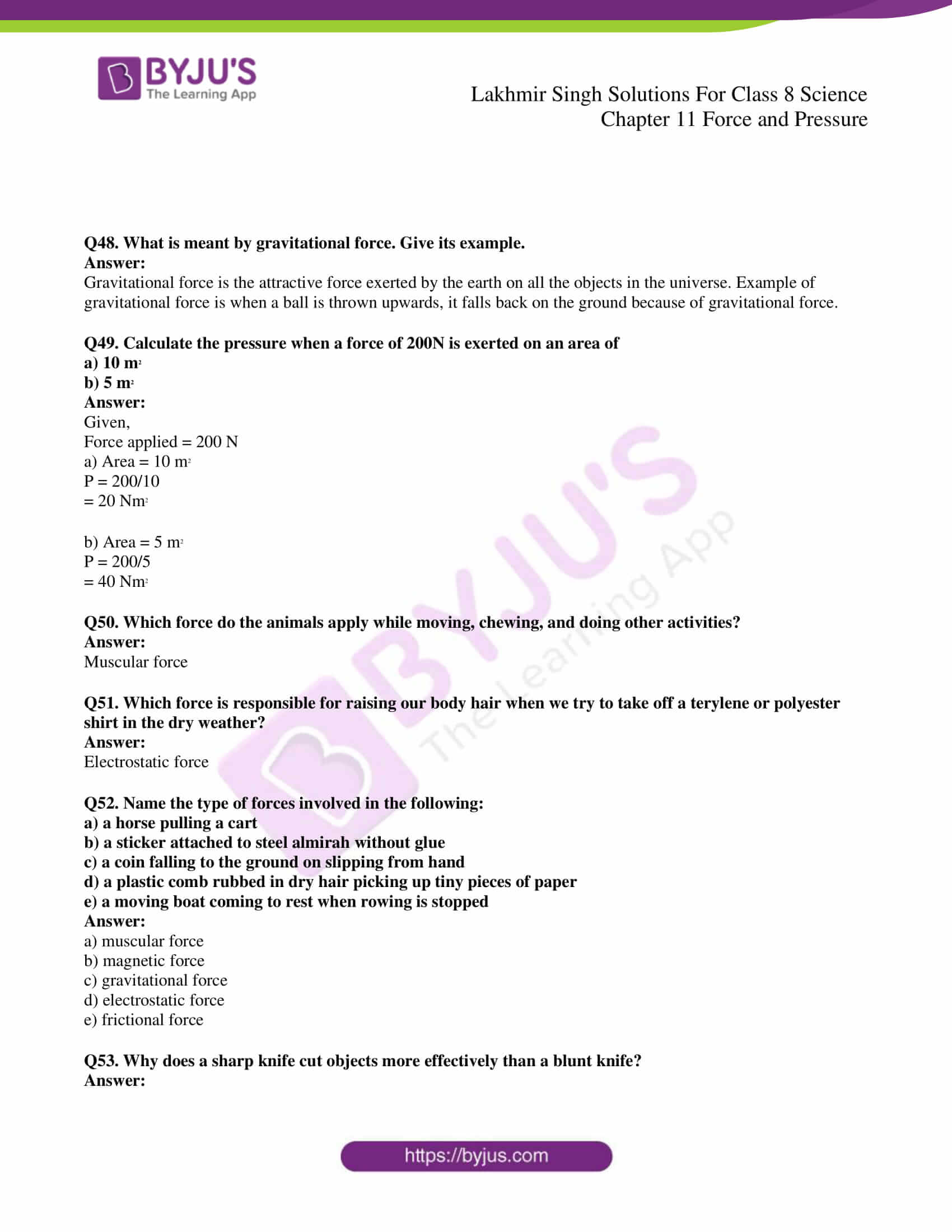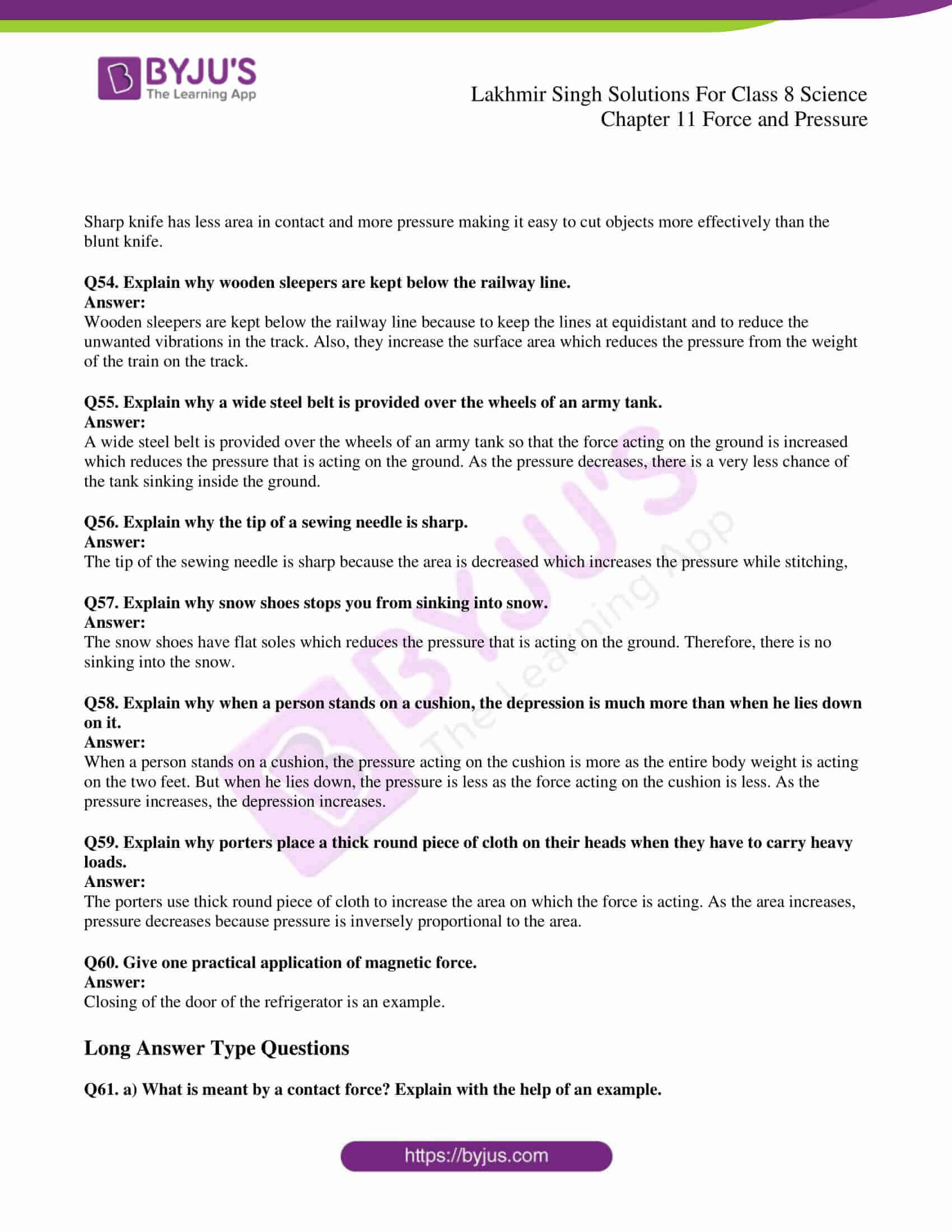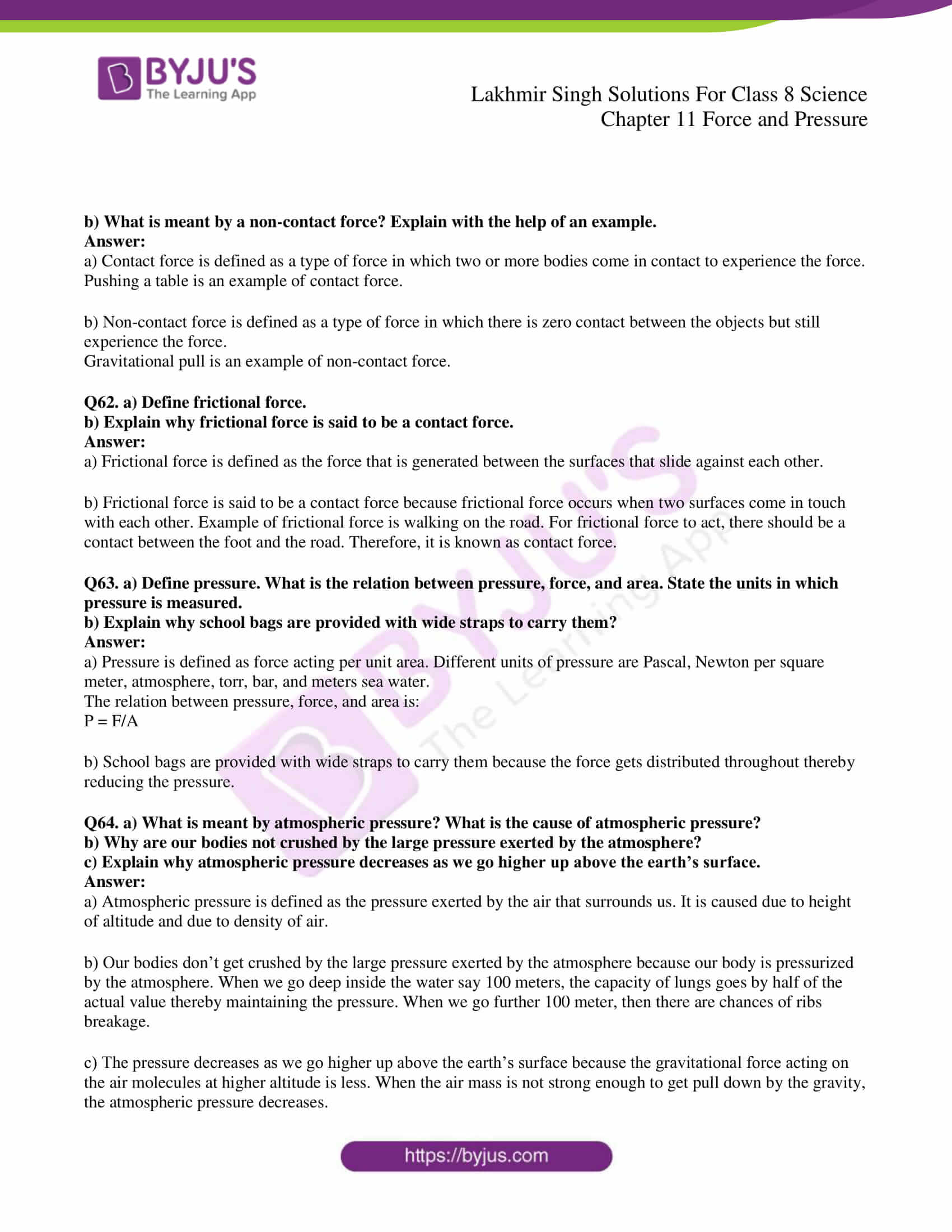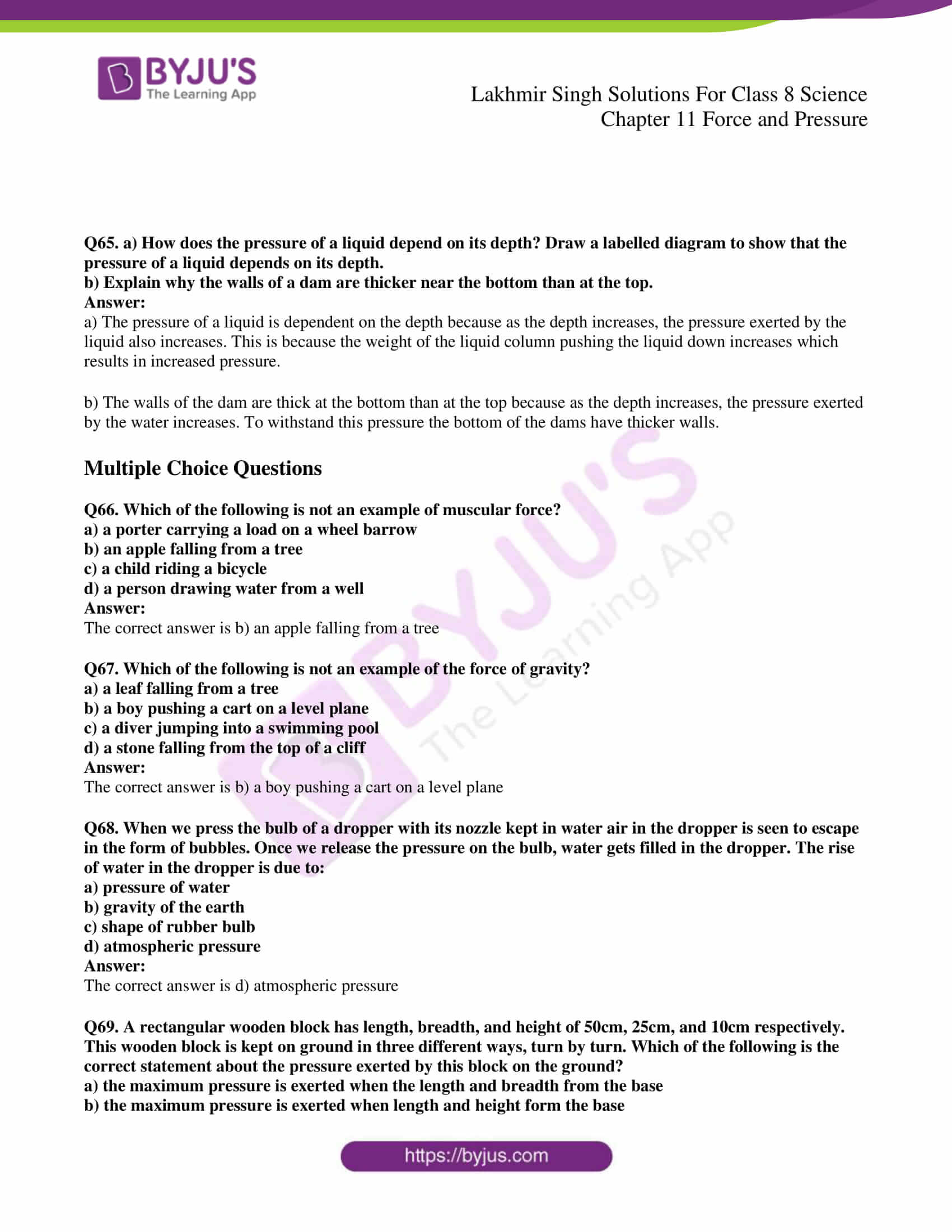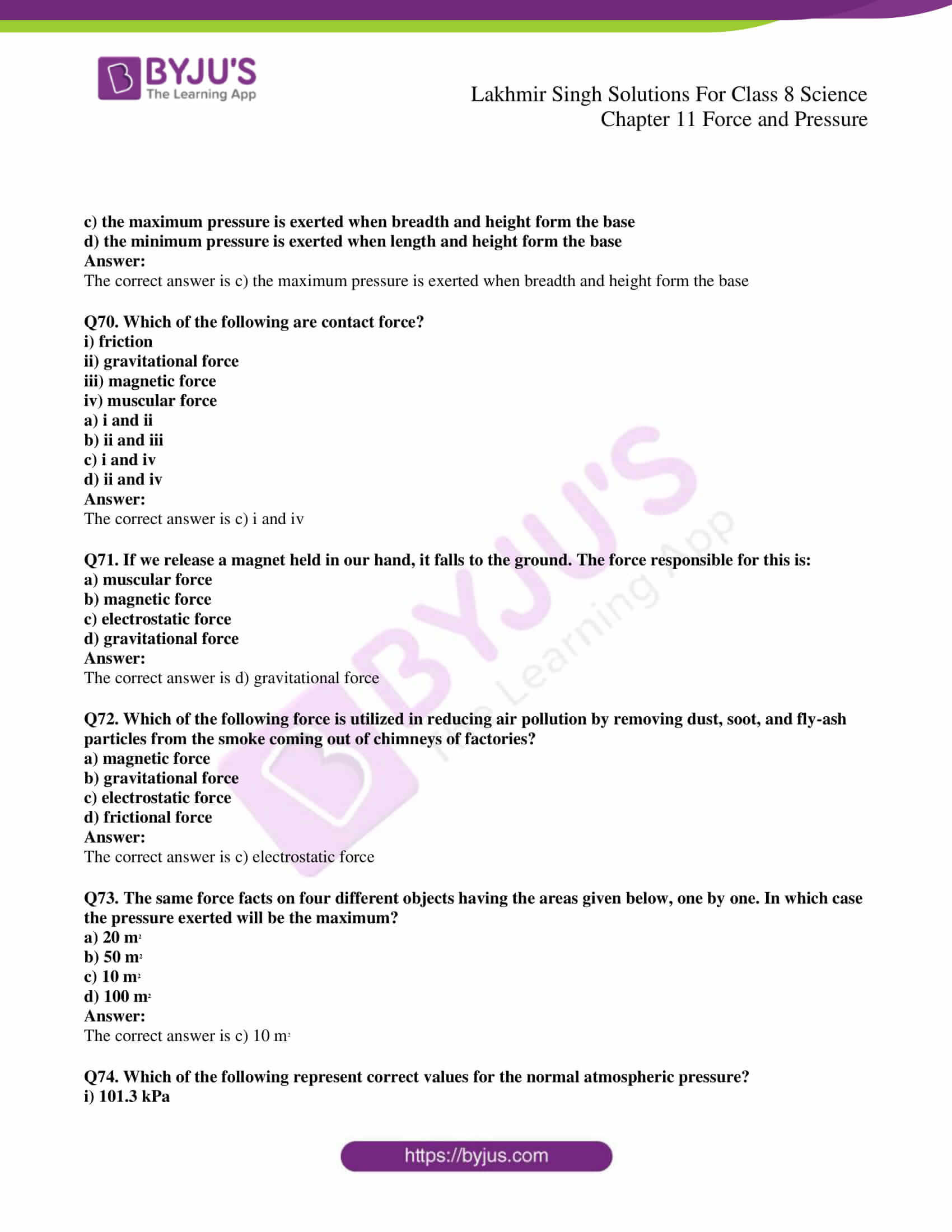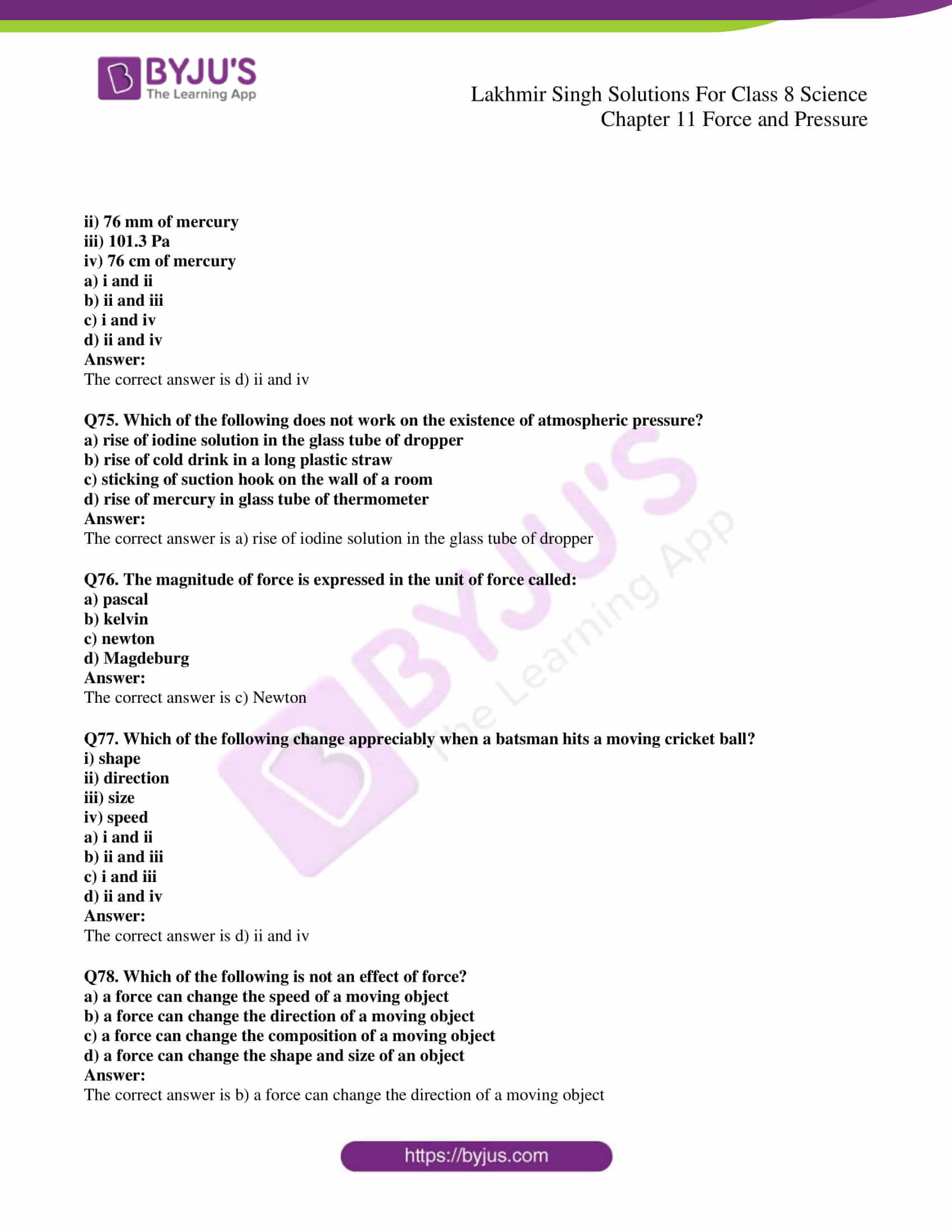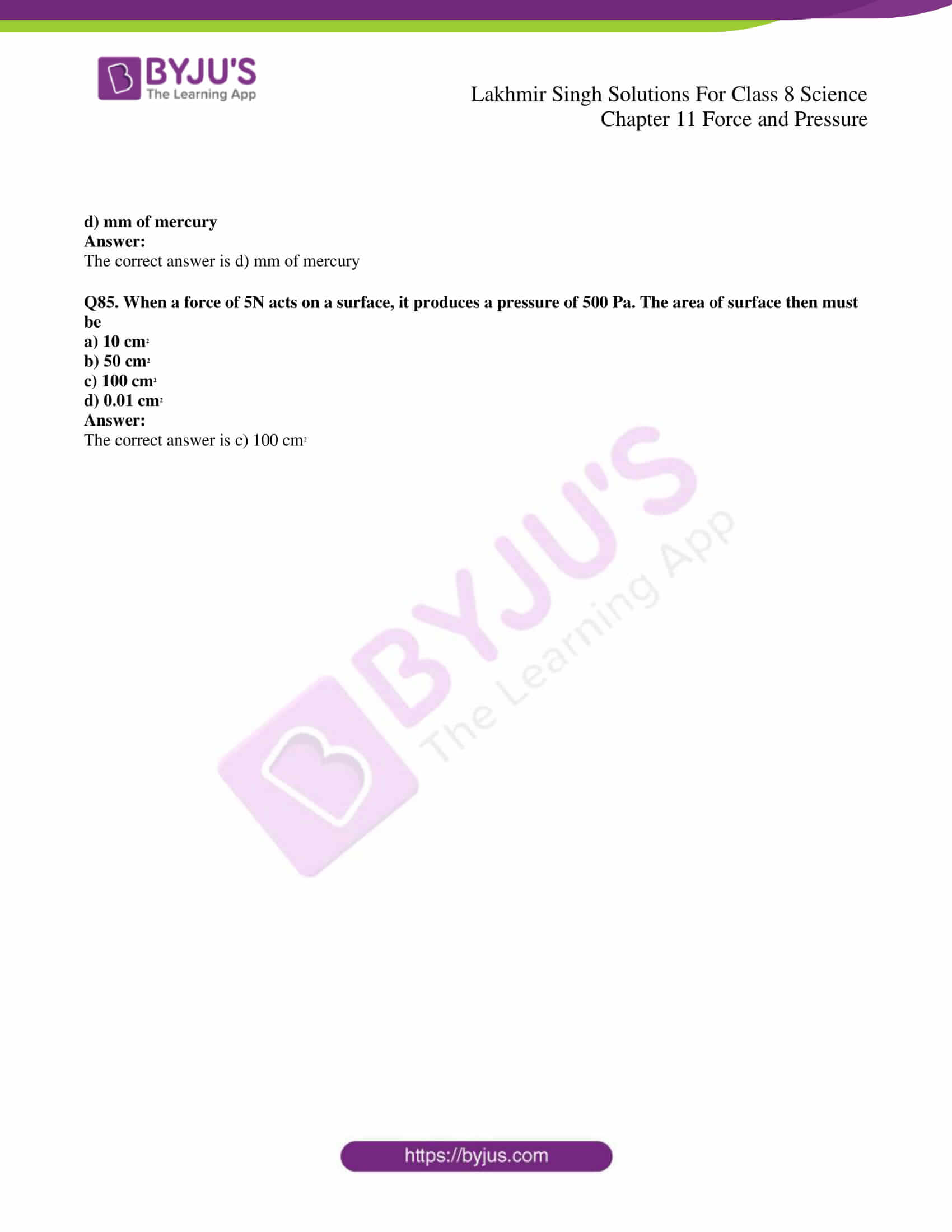### Very Short Answer Type Questions

Q1. What is the push or pull on an object known as?

The push and pull on an object is known as force.

Q2. Why do the shape and size of a balloon change when filled with air or water?

The shape and size of the balloon change when filled with air or water because they occupy space resulting in change in shape.

Q3. Name the quantity whose unit is Newton.

Newton is a unit of force.

Q4. When a ball is dropped from a height, its speed increases gradually. Name the force which causes this change in speed.

Gravitational force acts on the ball. Every object in the universe is affected by the gravitational force.

Q5. What is the unit of force?

Newton

Q6. Give one example where force changes the shape of an object.

The flattening of dough ball into a thin sheet with the help of rolling pin.

Q7. Identify the actions involved in the following situations as push or pull or both:

a) opening a drawer

b) a cricket ball hit by a batsman

c) drawing a bucket of water from a well

d) moving a book placed on a table

e) a football player taking a penalty corner

f) moving a wheel barrow

a) Pull

b) Push

c) Pull

d) Both

e) Push

f) Push

Q8. a) Name two contact forces

b) Name two non-contact forces

a) Frictional and applied forces

b) Gravitational and magnetic forces

Q9. When a plastic pen is rubbed in dry hair, it attracts tiny piece of paper. Which force is involved in this process?

Electrostatic force

Q10. A small device pulls iron nails from a distance. Which type of force is involved in this process?

Magnetic force

Q11. Which force can be used to gather iron pins scattered on the floor?

Magnetic force

Q12. Name the force which opposes the motion.

Frictional force

Q13. Which force makes a rolling ball stop on its own?

Frictional force

Q14. An inflated balloon was pressed against a wall after it has been rubbed with a piece of synthetic cloth. It was found that the balloon sticks to the wall. What force might be responsible for the attraction between the balloon and the wall?

Electrostatic force

Q15. What name is given to the force acting on a unit area of an object?

Pressure

Q16. Name the quantity whose one of the units is Pascal?

Pressure

Q17. What conclusion do you get from the observation that a fountain of water is created at the leaking joint of pipes of the main water supply line?

The conclusion drawn is that water exerts pressure at the bottom of the container. The fountain of water is created at the leaking joint of tubes of the main water supply line. The pressure is accomplished on the small opening of the pipe of the main water supply that drives the water to move out of the pipe by the pressure and hence create a fountain.

Q18. What type of pressure is involved in the filling of a liquid in a syringe?

Air pressure

Q19. What substance present in our body balances the atmospheric pressure acting on us?

Blood

Q20. Where will the atmospheric pressure be greater: at ground level or at the top of high mountain?

The atmospheric pressure will be more at the ground level.

Q21. Name any two devices used in everyday life which work on the existence of atmospheric pressure.

a) Vacuum cleaner

b) Drinking straw

Q22. If a vacuum is created between two Magdeburg hemispheres joined together, they cannot be separated easily. What presses the hemispheres together?

The two hemispheres stick to each other because the air inside them is pumped out with a remarkable force between them so that they can stick together.

Q23. What makes a balloon get inflated when air is filled in it?

A balloon gets inflated when air is filled in it because the pressure within the balloon increases and leading to increasing the surface area of the balloon. This provides high air pressure on the walls causing the balloon to expand and get inflated.

Q24. Name the substance whose weight produces atmospheric pressure.

The substance whose weight produces atmospheric pressure is air.

Q25. Where is the pressure greater, 10 m below the surface of the sea or 20 m below the surface of sea?

20 m below the surface of sea

Q26. What force acting on an area of 0.5 m2 will produce a pressure of 500 Pa?

P = F/A

F = PA

= 250 N

Q27. Can a liquid exert pressure upwards?

Yes, liquid can exert pressure upwards.

Q28. Can a liquid exert pressure sideways?

Yes, liquid can exert pressure sideways.

Q29. State whether the following statements are true or false:

a) The pressure exerted by a liquid depends on the area of base of its container.

b) A drinking straw works on the pressure exerted by the liquid filled in a soft drink bottle in which it is placed.

a) True

b) False

Q30. Fill in the following blanks with suitable words:

a) To draw water from a well, we have to …….. at the rope.

b) If the two forces applied to an object are equal and act in opposite directions, the net force acting on the object will be ……….

c) Force could be a ………. or a …………

d) Force has magnitude as well as ………….

e) A force arises due to …….. between two objects.

f) A charged body ……….. an unchanged body towards it.

g) The north pole of a magnet ………. the north pole of another magnet.

h) Force acting on a unit area is called …………

i) The pressure exerted by a liquid ……….. with depth.

j) A drinking straw works on the existence of ………. psressure.

k) Atmospheric pressure ……… with increasing height.

a) Pull

b) Zero

c) Push or pull

d) Direction

e) Interaction

f) Attracts

g) Repeals

h) Pressure

i) Increases

j) Atmospheric

k) Decreases

Q31. Define ‘state of motion’ of an object. Name the agent which can change the state of motion of an object.

The state of motion of an object is defined as the speed which is acquired by the object in a particular direction. Force is responsible for the change in the state of motion of an object.

Q32. Give two examples of situations where you push or pull to change the state of motion of objects.

a) Pushing a toy car forward

b) Sliding book on the table

Q33. What is meant by saying that force is due to an interaction? Give an example to illustrate your answer.

Force is due to an interaction means that force can exist only when two objects come in contact with each other. Example is moving the carom coin with a striker which results in the motion of the coin.

Q34. In a tug of war, when the two teams are pulling the rope, a stage comes when the rope does not move to either side at all. What can you say about the magnitudes and directions of the forces being applied to the rope by the two teams at this stage?

This happens when both the teams are applying equal amount of force such that the force from both the sides get cancelled.

Q35. What is force? State the various effects of force.

A force is defined as the push or pull experience by an object. Following are the various effects of force:

a) Results in change of shape

b) Results in change of direction

c) Results in change in position

Q36. a) Give one example where force moves a stationary object.

b) State one example where force stops a moving object.

a) Pushing a box

b) Applying brakes of car

Q37. a) Give one example where force changes the speed of a moving object.

b) Give one example where force changes the direction of a moving object.

a) Paddling more results in change in speed of a bicycle

b) Hitting a cricket ball changes the direction of the ball

Q38. Why does the shape of an ointment tube change when we squeeze it?

The shape of an ointment tube change when we squeeze it because force is applied to remove the ointment from the tube.

Q39. What happens to the springs of a sofa when we sit on it?

The shape and size of the springs of a sofa changes when we sit on it because force is applied on them.

Q40. Name the various types of forces.

The various types of forces are:

Magnetic force, frictional force, applied force, spring force, electrical force, gravitational force, tension force, normal force, and air resistance force.

Q41. What is muscular force? Give one example of muscular force.

Muscular force refers to the force which is produced by the muscles during heavy workouts which results in the production of the lactic acid. Muscular force is used for bouncing a ball.

Q42. Which of the following are non-contact forces?

Magnetic force, frictional force, gravitational force, muscular force, electrostatic force.

Magnetic force, gravitational force, and electrostatic force.

Q43. Give two examples from everyday life which show that air exerts pressure.

Vacuum cleaner and drinking from straw are the examples.

Q44. What is a rubber sucker? How does it work? State any one use of a rubber sucker.

Rubber sucker is a device which is used in place of glue for a temporary period which is used as sticking device.

The principle behind the working of a rubber sucker is that when it is pressed against the wall or any surface it squeezes the air such that the atmospheric pressure outside the sucker holds it firmly against the surface.

Q45. Why do mountaineers usually suffer from nose-bleeding at high altitude?

They suffer from nose-bleeding at high altitudes because the atmospheric pressure decreases and the blood vessels get raptured as the pressure inside increases.

Q46. Describe one activity to show the existence of atmospheric pressure.

The crushing of a plastic bottle when filled with hot water is an example of existence of atmospheric pressure.

Q47. Explain why water comes out more slowly from an upstairs tap than from a similar tap downstairs.

This is because the depth of the water increases making the pressure increase too.

Q48. What is meant by gravitational force. Give its example.

Gravitational force is the attractive force exerted by the earth on all the objects in the universe. Example of gravitational force is when a ball is thrown upwards, it falls back on the ground because of gravitational force.

Q49. Calculate the pressure when a force of 200N is exerted on an area of

a) 10 m2

b) 5 m2

Given,

Force applied = 200 N

a) Area = 10 m2

P = 200/10

= 20 Nm2

b) Area = 5 m2

P = 200/5

= 40 Nm2

Q50. Which force do the animals apply while moving, chewing, and doing other activities?

Muscular force

Q51. Which force is responsible for raising our body hair when we try to take off a terylene or polyester shirt in the dry weather?

Electrostatic force

Q52. Name the type of forces involved in the following:

a) a horse pulling a cart

b) a sticker attached to steel almirah without glue

c) a coin falling to the ground on slipping from hand

d) a plastic comb rubbed in dry hair picking up tiny pieces of paper

e) a moving boat coming to rest when rowing is stopped

a) muscular force

b) magnetic force

c) gravitational force

d) electrostatic force

e) frictional force

Q53. Why does a sharp knife cut objects more effectively than a blunt knife?

Sharp knife has less area in contact and more pressure making it easy to cut objects more effectively than the blunt knife.

Q54. Explain why wooden sleepers are kept below the railway line.

Wooden sleepers are kept below the railway line because to keep the lines at equidistant and to reduce the unwanted vibrations in the track. Also, they increase the surface area which reduces the pressure from the weight of the train on the track.

Q55. Explain why a wide steel belt is provided over the wheels of an army tank.

A wide steel belt is provided over the wheels of an army tank so that the force acting on the ground is increased which reduces the pressure that is acting on the ground. As the pressure decreases, there is a very less chance of the tank sinking inside the ground.

Q56. Explain why the tip of a sewing needle is sharp.

The tip of the sewing needle is sharp because the area is decreased which increases the pressure while stitching,

Q57. Explain why snow shoes stops you from sinking into snow.

The snow shoes have flat soles which reduces the pressure that is acting on the ground. Therefore, there is no sinking into the snow.

Q58. Explain why when a person stands on a cushion, the depression is much more than when he lies down on it.

When a person stands on a cushion, the pressure acting on the cushion is more as the entire body weight is acting on the two feet. But when he lies down, the pressure is less as the force acting on the cushion is less. As the pressure increases, the depression increases.

Q59. Explain why porters place a thick round piece of cloth on their heads when they have to carry heavy loads.

The porters use thick round piece of cloth to increase the area on which the force is acting. As the area increases, pressure decreases because pressure is inversely proportional to the area.

Q60. Give one practical application of magnetic force.

Closing of the door of the refrigerator is an example.

Q61. a) What is meant by a contact force? Explain with the help of an example.

b) What is meant by a non-contact force? Explain with the help of an example.

a) Contact force is defined as a type of force in which two or more bodies come in contact to experience the force. Pushing a table is an example of contact force.

b) Non-contact force is defined as a type of force in which there is zero contact between the objects but still experience the force.

Gravitational pull is an example of non-contact force.

Q62. a) Define frictional force.

b) Explain why frictional force is said to be a contact force.

a) Frictional force is defined as the force that is generated between the surfaces that slide against each other.

b) Frictional force is said to be a contact force because frictional force occurs when two surfaces come in touch with each other. Example of frictional force is walking on the road. For frictional force to act, there should be a contact between the foot and the road. Therefore, it is known as contact force.

Q63. a) Define pressure. What is the relation between pressure, force, and area. State the units in which pressure is measured.

b) Explain why school bags are provided with wide straps to carry them?

a) Pressure is defined as force acting per unit area. Different units of pressure are Pascal, Newton per square meter, atmosphere, torr, bar, and meters sea water.

The relation between pressure, force, and area is:

P = F/A

b) School bags are provided with wide straps to carry them because the force gets distributed throughout thereby reducing the pressure.

Q64. a) What is meant by atmospheric pressure? What is the cause of atmospheric pressure?

b) Why are our bodies not crushed by the large pressure exerted by the atmosphere?

c) Explain why atmospheric pressure decreases as we go higher up above the earth’s surface.

a) Atmospheric pressure is defined as the pressure exerted by the air that surrounds us. It is caused due to height of altitude and due to density of air.

b) Our bodies don’t get crushed by the large pressure exerted by the atmosphere because our body is pressurized by the atmosphere. When we go deep inside the water say 100 meters, the capacity of lungs goes by half of the actual value thereby maintaining the pressure. When we go further 100 meter, then there are chances of ribs breakage.

c) The pressure decreases as we go higher up above the earth’s surface because the gravitational force acting on the air molecules at higher altitude is less. When the air mass is not strong enough to get pull down by the gravity, the atmospheric pressure decreases.

Q65. a) How does the pressure of a liquid depend on its depth? Draw a labelled diagram to show that the pressure of a liquid depends on its depth.

b) Explain why the walls of a dam are thicker near the bottom than at the top.

a) The pressure of a liquid is dependent on the depth because as the depth increases, the pressure exerted by the liquid also increases. This is because the weight of the liquid column pushing the liquid down increases which results in increased pressure.

b) The walls of the dam are thick at the bottom than at the top because as the depth increases, the pressure exerted by the water increases. To withstand this pressure the bottom of the dams have thicker walls.

Multiple Choice Questions

Q66. Which of the following is not an example of muscular force?

a) a porter carrying a load on a wheel barrow

b) an apple falling from a tree

c) a child riding a bicycle

d) a person drawing water from a well

The correct answer is b) an apple falling from a tree

Q67. Which of the following is not an example of the force of gravity?

a) a leaf falling from a tree

b) a boy pushing a cart on a level plane

c) a diver jumping into a swimming pool

d) a stone falling from the top of a cliff

The correct answer is b) a boy pushing a cart on a level plane

Q68. When we press the bulb of a dropper with its nozzle kept in water air in the dropper is seen to escape in the form of bubbles. Once we release the pressure on the bulb, water gets filled in the dropper. The rise of water in the dropper is due to:

a) pressure of water

b) gravity of the earth

c) shape of rubber bulb

d) atmospheric pressure

The correct answer is d) atmospheric pressure

Q69. A rectangular wooden block has length, breadth, and height of 50cm, 25cm, and 10cm respectively. This wooden block is kept on ground in three different ways, turn by turn. Which of the following is the correct statement about the pressure exerted by this block on the ground?

a) the maximum pressure is exerted when the length and breadth from the base

b) the maximum pressure is exerted when length and height form the base

c) the maximum pressure is exerted when breadth and height form the base

d) the minimum pressure is exerted when length and height form the base

The correct answer is c) the maximum pressure is exerted when breadth and height form the base

Q70. Which of the following are contact force?

i) friction

ii) gravitational force

iii) magnetic force

iv) muscular force

a) i and ii

b) ii and iii

c) i and iv

d) ii and iv

The correct answer is c) i and iv

Q71. If we release a magnet held in our hand, it falls to the ground. The force responsible for this is:

a) muscular force

b) magnetic force

c) electrostatic force

d) gravitational force

The correct answer is d) gravitational force

Q72. Which of the following force is utilized in reducing air pollution by removing dust, soot, and fly-ash particles from the smoke coming out of chimneys of factories?

a) magnetic force

b) gravitational force

c) electrostatic force

d) frictional force

The correct answer is c) electrostatic force

Q73. The same force facts on four different objects having the areas given below, one by one. In which case the pressure exerted will be the maximum?

a) 20 m2

b) 50 m2

c) 10 m2

d) 100 m2

The correct answer is c) 10 m2

Q74. Which of the following represent correct values for the normal atmospheric pressure?

i) 101.3 kPa

ii) 76 mm of mercury

iii) 101.3 Pa

iv) 76 cm of mercury

a) i and ii

b) ii and iii

c) i and iv

d) ii and iv

The correct answer is d) ii and iv

Q75. Which of the following does not work on the existence of atmospheric pressure?

a) rise of iodine solution in the glass tube of dropper

b) rise of cold drink in a long plastic straw

c) sticking of suction hook on the wall of a room

d) rise of mercury in glass tube of thermometer

The correct answer is a) rise of iodine solution in the glass tube of dropper

Q76. The magnitude of force is expressed in the unit of force called:

a) pascal

b) kelvin

c) newton

d) Magdeburg

The correct answer is c) Newton

Q77. Which of the following change appreciably when a batsman hits a moving cricket ball?

i) shape

ii) direction

iii) size

iv) speed

a) i and ii

b) ii and iii

c) i and iii

d) ii and iv

The correct answer is d) ii and iv

Q78. Which of the following is not an effect of force?

a) a force can change the speed of a moving object

b) a force can change the direction of a moving object

c) a force can change the composition of a moving object

d) a force can change the shape and size of an object

The correct answer is b) a force can change the direction of a moving object

Q79. Which of the following is not a non-contact force?

a) electrostatic force

b) gravitational force

c) frictional force

d) magnetic force

The correct answer is b) gravitational force

Q80. Which of the following scientists gave the idea of the existence of gravitational force?

a) Einstein

b) James Watt

d) Newton

The correct answer is d) Newton

Q81. Some mustard oil is kept in a beaker. It will exert pressure:

a) downwards only

b) sideways only

c) upwards only

d) in all directions

The correct answer is d) in all directions

Q82. A pressure of 10 kPa acts on an area of 0.3m2. The force acting on the area will be:

a) 3000 N

b) 30 N

c) 3 N

d) 300 N

The correct answer is a) 3000 N

Q83. The magnitude of atmospheric pressure is equal to the pressure exerted by a:

a) 76 mm tall column of mercury

b) 760 mm tall column of alcohol

c) 76 cm tall column of mercury

d) 760 cm tall column of mercury

The correct answer is c) 76 cm tall column of mercury

Q84. The atmospheric pressure is usually measured in the unit of:

a) Newtons per square meter

b) Pascal

c) cm of mercury

d) mm of mercury

The correct answer is d) mm of mercury

Q85. When a force of 5N acts on a surface, it produces a pressure of 500 Pa. The area of surface then must be

a) 10 cm2

b) 50 cm2

c) 100 cm2

d) 0.01 cm2

The correct answer is c) 100 cm2

Also, visit Lakhmir Singh Solutions for Class 8 Science to get complete solutions for all chapters.

Lakhmir Singh Class 8 Science Solutions Chapter 11, Lakhmir Singh Science Solutions Class 8 Chapter 11, Lakhmir Singh Solutions Class 9 Biology Chapter 8 Science Chapter 11 Force and Pressure PDF

1. Arpita Singh

Nice description. Very happy to read. Thanks for guidelines provided by you all.

2. Tapas Kumar Jana

Excellent

3. Tapas Kumar Jana

Excellent

4. Dipam Mukherjee

Excellent guys